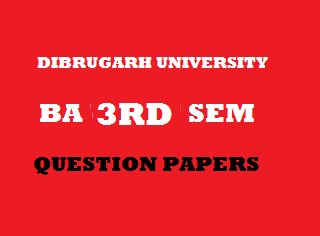## Wednesday, January 02, 20192013
(November)
EDUCATION
(General)
Course: 301
(Measurement and Evaluation in Education)
Full Marks: 80
Pass Marks: 32
Time: 3 hours
The figures in the margin indicate full marks for the questions

1. Give short answers to the following questions: 1x8=8
1. Mention one difference between measurement and evaluation.
2. What is meant by test?
3. Write True or False:
If the number of items in a test increases, the reliability of the test also increases.
1. Cite an example of a group test.
2. Who introduced the term ‘Intelligence Quotient’?
3. If the chronological age of a student is 16 and mental age is 14, what will be his IQ?
4. Who introduced the inkblot test of measuring personality/
5. Mention a merit of mean as a measure of central tendency.
2. What is meant by evaluation? Explain the concept of comprehensive evaluation. Discuss its application in the classroom. 10
3. What is meant by validity? Describe the different types of validity. 4+6=10
Or
Describe the process of standardization of an achievement test. 10
4. What is meant by projective technique? Discuss the Thematic Apperception Test. (TAT) in detail.
4+6=10
Or
What is meant by aptitude test? What are the different types of aptitude test? What are the uses of aptitude test? 3+3+4=10
5. Write short notes on the following (any three): 5x3=15
1. Need measurement in education.
2. Objectivity of a test.
3. Observation.
4. Scope of Educational Statistics.
6. What is meant by central tendency? State the merits and demerits of median as a measure of central tendency? 2+3+3=8
7. Find out mean from the following frequency distribution table: 8
 Class Interval Frequency 65-6960-6455-5950-5445-4940-4435-3930-3425-2920-24 13479118421 N = 50

8. Draw a histogram from the following frequency distribution table:
 Class Interval Frequency 60-6455-5950-5445-4940-4435-3930-3425-2920-24 345678412 N = 40

9. Determine the correlation coefficient by rank difference method from the following two sets of scores. 7
 Roll No.: 1 2 3 4 5 6 7 8 X: 15 18 22 17 19 20 16 21 Y: 40 42 50 45 43 46 41 41

***